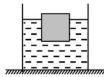# 如图,将一边长为10 cm的正方体木块放入装有某液体的圆柱形容器中。木块静止时1条回答
• 答案：640  6.4

解析：

设液体的密度是ρ，则

p=ρgh

ρ=p/(gh)

=80Pa/(10N/kg×1×10-2m)

=0.8×103kg/m3

物体浸入液体中的体积：、

V=(10cm)2×(10cm-2cm)

=800cm3

=8×10-4m3

浮力大小

F=ρgV

=0.8×103kg/m3×10N/kg×8×10-4m3

=6.4N

木块底部受到的压力

F=F=6.4N

其压强大小是

p=F/S

=6.4N/(10×10×10-4m2)

=640Pa

讨论(0)看不清?